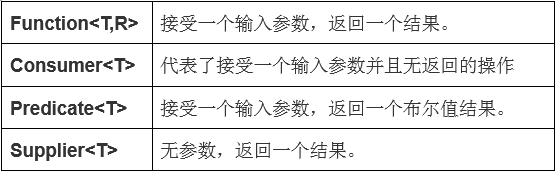# JDK8函数式接口Function、Consumer、Predicate、Supplier

4 篇文章 0 订阅1、三种方法
1.1 唯一的抽象方法
1.2 使用default定义普通方法（默认方法），通过对象调用。

3.使用static定义静态方法，通过接口名调用。
2、一个新注解

JDK8以前，通过匿名内部类可以实现接口

        Function<Integer, String> fun = new Function<Integer, String>() {
@Override
public String apply(Integer t) {
return String.valueOf(t);
}
};

JDK8中，通过lambda表达式实现

Function<Integer, String> fun = (x) -> String.valueOf(x);

String res = fun.apply(1000);
System.out.println(res); 

## 一、Function功能型函数式接口

Function接口 接受一个输入参数T，返回一个结果R。

package java.util.function;
import java.util.Objects;

@FunctionalInterface
public interface Function<T, R> {
// 接受输入参数，对输入执行所需操作后  返回一个结果。
R apply(T t);

// 返回一个 先执行before函数对象apply方法，再执行当前函数对象apply方法的 函数对象。
default <V> Function<V, R> compose(Function<? super V, ? extends T> before) {
Objects.requireNonNull(before);
return (V v) -> apply(before.apply(v));
}

// 返回一个 先执行当前函数对象apply方法， 再执行after函数对象apply方法的 函数对象。
default <V> Function<T, V> andThen(Function<? super R, ? extends V> after) {
Objects.requireNonNull(after);
return (T t) -> after.apply(apply(t));
}

// 返回一个执行了apply()方法之后只会返回输入参数的函数对象。
static <T> Function<T, T> identity() {
return t -> t;
}
}

public class FunctionDemo {

static int modifyTheValue(int valueToBeOperated, Function<Integer, Integer> function) {
return function.apply(valueToBeOperated);
}

public static void main(String[] args) {
int myNumber = 10;

// 使用lambda表达式实现函数式接口
// (x)->(x)+20 输入一个参数x，进行加法运算，返回一个结果
// 所以该lambda表达式可以实现Function接口
int res1 = modifyTheValue(myNumber, (x)-> x + 20);
System.out.println(res1); // 30

//  使用匿名内部类实现
int res2 = modifyTheValue(myNumber, new Function<Integer, Integer>() {
@Override
public Integer apply(Integer t) {
return t + 20;
}
});
System.out.println(res2); // 30
}
}

    public static Integer modifyTheValue2(int value, Function<Integer, Integer> function1, Function<Integer, Integer> function2){
//value作为function1的参数，返回一个结果，该结果作为function2的参数，返回一个最终结果
return  function1.andThen(function2).apply(value);
}

public static void main(String[] args) {
System.out.println(modifyTheValue2(3, val -> val + 2, val -> val + 3));
}

## 二、Consumer消费型函数式接口

    public static void modifyTheValue3(int value, Consumer<Integer> consumer) {
consumer.accept(value);
}

public static void main(String[] args) {
// (x) -> System.out.println(x * 2)接受一个输入参数x
// 直接输出，并没有返回结果
// 所以该lambda表达式可以实现Consumer接口
modifyTheValue3(3, (x) -> System.out.println(x * 2));
}

6

## 三、Predicate断言型函数式接口

    public static boolean predicateTest(int value, Predicate<Integer> predicate) {
return predicate.test(value);
}

public static void main(String[] args) {
// (x) -> x == 3 输入一个参数x，进行比较操作，返回一个布尔值
// 所以该lambda表达式可以实现Predicate接口
System.out.println(predicateTest(3, (x) -> x == 3));
}

true

    public static void eval(List<Integer> list, Predicate<Integer> predicate) {
for (Integer n : list) {
if (predicate.test(n)) {
System.out.print(n + " ");
}
}

//      list.forEach(n -> {
//          if (predicate.test(n)) {
//              System.out.print(n + " ");
//          }
//      });
}

public static void main(String args[]) {
List<Integer> list = Arrays.asList(1, 2, 3, 4, 5, 6, 7, 8, 9);

// Predicate<Integer> predicate = n -> true
// n 是一个参数传递到 Predicate 接口的 test 方法
// n 如果存在则 test 方法返回 true

System.out.println("输出所有数据:");

// 传递参数 n
eval(list, n -> true);

// Predicate<Integer> predicate1 = n -> n%2 == 0
// n 是一个参数传递到 Predicate 接口的 test 方法
// 如果 n%2 为 0 test 方法返回 true

System.out.println("\n输出所有偶数:");
eval(list, n -> n % 2 == 0);

// Predicate<Integer> predicate2 = n -> n > 3
// n 是一个参数传递到 Predicate 接口的 test 方法
// 如果 n 大于 3 test 方法返回 true

System.out.println("\n输出大于 3 的所有数字:");
eval(list, n -> n > 3);
}

输出所有数据:
1 2 3 4 5 6 7 8 9

2 4 6 8

4 5 6 7 8 9 

    public static boolean validInput(String name, Predicate<String> function) {
return function.test(name);
}

public static void main(String args[]) {
String name = "冷冷";
if(validInput(name, s -> !s.isEmpty() &&  s.length() <= 3 )) {
System.out.println("名字输入正确");
}
}

## 三、Supplier供给型函数式接口

    public static String supplierTest(Supplier<String> supplier) {
return supplier.get();
}

public static void main(String args[]) {
String name = "冷冷";
// () -> name.length() 无参数，返回一个结果（字符串长度）
// 所以该lambda表达式可以实现Supplier接口
System.out.println(supplierTest(() -> name.length() + ""));
}

2
01-09
01-10229409-06687
09-032146
01-137145
08-291134
05-13480
06-10627
11-16616
04-15
06-03
03-193万+
06-162020
01-061579
11-11203
11-17715点击重新获取扫码支付余额充值